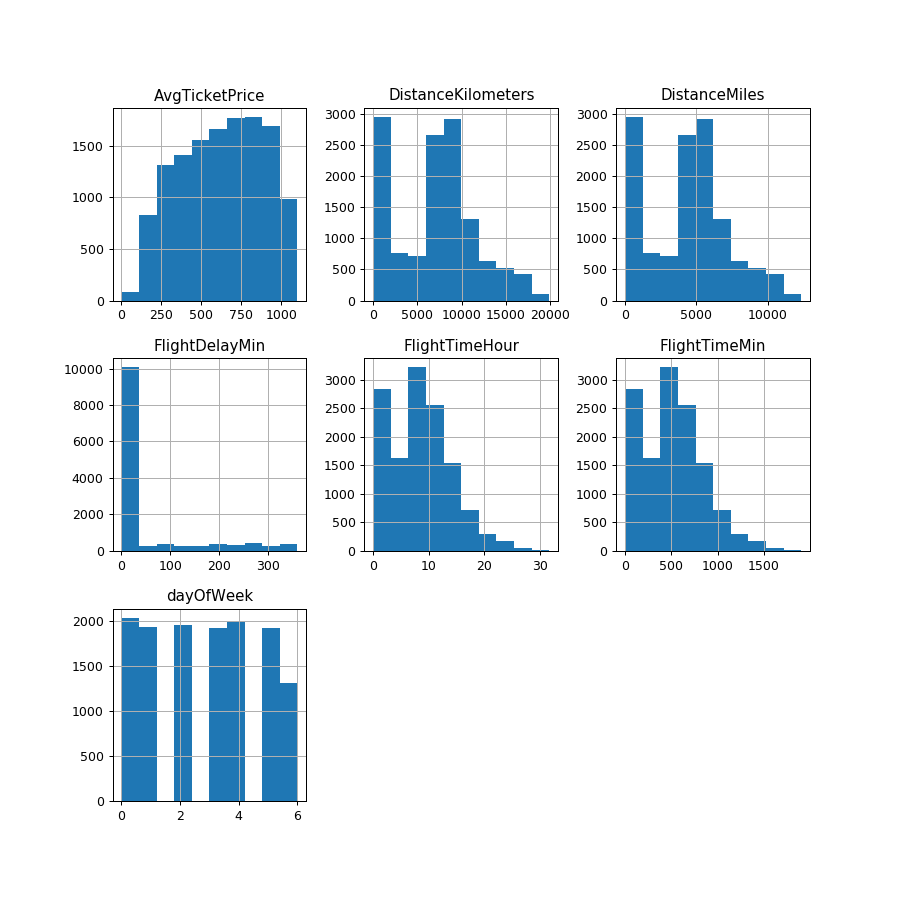# eland.DataFrame.hist¶

DataFrame.hist(column=None, by=None, grid=True, xlabelsize=None, xrot=None, ylabelsize=None, yrot=None, ax=None, sharex=False, sharey=False, figsize=None, layout=None, bins=10, **kwds)

Make a histogram of the DataFrame’s.

See pandas.DataFrame.hist for usage.

Notes

Derived from pandas.plotting._core.hist_frame 0.25.3

Ideally, we’d call the pandas method hist_frame directly with histogram data, but weights are applied to ALL series. For example, we can plot a histogram of pre-binned data via:

counts, bins = np.histogram(data)
plt.hist(bins[:-1], bins, weights=counts)


However,

ax.hist(data[col].dropna().values, bins=bins, **kwds)


is for [col] and weights are a single array.

Examples

>>> df = ed.DataFrame('localhost', 'flights')
>>> hist = df.select_dtypes(include=[np.number]).hist(figsize=[10,10]) # doctest: +SKIP